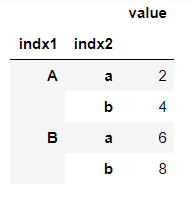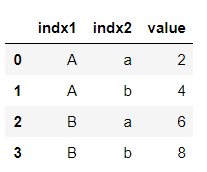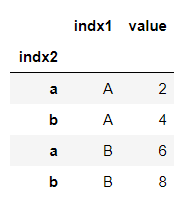# Turn Pandas Multi-Index into column

• Last Updated : 06 Oct, 2021

Pandas DataFrame is a two-dimensional size-mutable, potentially heterogeneous tabular data structure with labeled axes (rows and columns). A multi-index dataframe has multi-level, or hierarchical indexing. We can easily convert the multi-level index into the column by the reset_index() method.

DataFrame.reset_index() is used to reset the index to default and make the index a column of the dataframe.

Step 1: Creating a multi-index dataframe.

Let’s see an example by making a multi-index dataframe first.

Code:

## Python3

 `import` `pandas as pd` `# Creating index for multi-index dataframe``tuples ``=` `[(``'A'``, ``'a'``), (``'A'``, ``'b'``), (``'B'``, ``'a'``), (``'B'``, ``'b'``)]``index ``=` `pd.MultiIndex.from_tuples(tuples)` `# Value corresponding to the index``data ``=` `[``2``, ``4``, ``6``, ``8``]` `# Creating dataframe using 'data' and 'index'``df ``=` `pd.DataFrame(data ``=` `data, index ``=` `index, columns ``=` `[``'value'``])``print``(df)`

Output:Step 2: Converting index into the column.

Here we can see the hierarchical indexing, we are going to convert it into a column using the reset_index() method.

## Python3

 `reset_df ``=` `df.reset_index()``print``(reset_df)`

Output:Here we can see that the index is default now and our multi-index now becomes columns with the default. We can also select which level of multi-index to reset using parameter level.

Code:

## Python3

 `# rest only index 'indx1'``reset_indx1 ``=` `df.reset_index(level``=``'indx1'``)``print``(reset_indx1)`

Output:Here, we can see that only indx1 is reset becomes a column, not both the indices. Also, notice that there is no default index in this case because there is still an index (indx2) left.

My Personal Notes arrow_drop_up